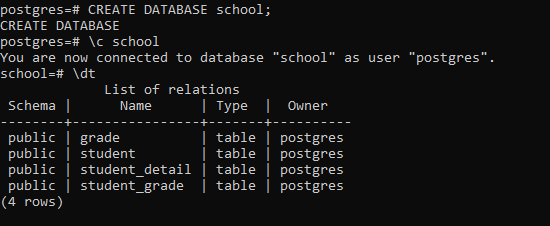Related Articles

# PostgreSQL – Create table using Python

• Last Updated : 30 Aug, 2020

This article explores the process of creating table in The PostgreSQL database using Python.

### Prerequisites:

• psycopg2 module
• sample database

### Creating a Table:

To create a table in the database use the following steps:

• First create a CREATE TABLE statement
• Second establish a connection to the database using the connect() function
• Third construct a cursor object by using the cursor() method.
• Now execute the above created CREATE TABLE statement using the execute function.

Example:

In this example we have already created a Database called school. We will be adding tables to it. To do so we created a file called create_table.py and defined a create_table() function as shown below:

## Python3

 `import` `psycopg2``from` `config ``import` `config`` ` ` ` `def` `create_tables():``    ``""" create tables in the PostgreSQL database"""``    ``commands ``=` `(``        ``"""``        ``CREATE TABLE student (``            ``student_id SERIAL PRIMARY KEY,``            ``student_name VARCHAR(255) NOT NULL``        ``)``        ``"""``,``        ``""" CREATE TABLE grade (``                ``grade_id SERIAL PRIMARY KEY,``                ``grade_name VARCHAR(255) NOT NULL``                ``)``        ``"""``,``        ``"""``        ``CREATE TABLE student_grade (``                ``grade_id INTEGER PRIMARY KEY,``                ``file_extension VARCHAR(5) NOT NULL,``                ``drawing_data BYTEA NOT NULL,``                ``FOREIGN KEY (grade_id)``                ``REFERENCES grade (grade_id)``                ``ON UPDATE CASCADE ON DELETE CASCADE``        ``)``        ``"""``,``        ``"""``        ``CREATE TABLE student_detail (``                ``student_id INTEGER NOT NULL,``                ``grade_id INTEGER NOT NULL,``                ``PRIMARY KEY (student_id , grade_id),``                ``FOREIGN KEY (student_id)``                    ``REFERENCES student (student_id)``                    ``ON UPDATE CASCADE ON DELETE CASCADE,``                ``FOREIGN KEY (grade_id)``                    ``REFERENCES grade (grade_id)``                    ``ON UPDATE CASCADE ON DELETE CASCADE``        ``)``        ``"""``)``    ``conn ``=` `None``    ``try``:``        ``# read the connection parameters``        ``params ``=` `config()``        ``# connect to the PostgreSQL server``        ``conn ``=` `psycopg2.connect(``*``*``params)``        ``cur ``=` `conn.cursor()``        ``# create table one by one``        ``for` `command ``in` `commands:``            ``cur.execute(command)``        ``# close communication with the PostgreSQL database server``        ``cur.close()``        ``# commit the changes``        ``conn.commit()``    ``except` `(Exception, psycopg2.DatabaseError) as error:``        ``print``(error)``    ``finally``:``        ``if` `conn ``is` `not` `None``:``            ``conn.close()`` ` ` ` `if` `__name__ ``=``=` `'__main__'``:``    ``create_tables()`

This will successfully create the tables :

• student
• student_detail

To verify so use the below command through the client tool of the same database(ie, school):

```\dt
```

Output:Attention geek! Strengthen your foundations with the Python Programming Foundation Course and learn the basics.

To begin with, your interview preparations Enhance your Data Structures concepts with the Python DS Course. And to begin with your Machine Learning Journey, join the Machine Learning – Basic Level Course

My Personal Notes arrow_drop_up